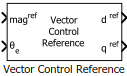# Vector Control Reference

Compute d and q axis components of reference vector

Since R2020a

•Libraries:
Motor Control Blockset / Controls / Control Reference

## Description

The Vector Control Reference block calculates the d-axis and q-axis components of the reference voltage, current, or flux vector that you provide as an input to the block.

The block accepts magnitude and position of the reference vector as inputs. The block outputs the reference vector components along the direct and quadrature axes of the rotating dq reference frame.

### Equations

The block uses these equations to compute the d-axis and q-axis vector component outputs.

`${d}^{ref}=ma{g}^{ref}×\text{cos}{\theta }_{e}$`
`${q}^{ref}=ma{g}^{ref}×\text{sin}{\theta }_{e}$`

where:

• ${d}^{ref}$ is the d-axis component of the reference vector.

• ${q}^{ref}$ is the q-axis component of the reference vector.

• $ma{g}^{ref}$ is the magnitude of the reference vector.

• ${\theta }_{e}$ is the electrical position of the reference vector.

## Ports

### Input

expand all

Magnitude of the reference voltage, current, or flux vector that you provide as an input to the block.

Data Types: `single` | `double` | `fixed point`

Electrical position of the reference voltage, current, or flux vector that you provide as an input to the block.

Data Types: `single` | `double` | `fixed point`

### Output

expand all

Reference voltage, current, or flux vector component along the direct axis of the rotating dq reference frame.

Data Types: `single` | `double` | `fixed point`

Reference voltage, current, or flux vector component along the quadrature axis of the rotating dq reference frame.

Data Types: `single` | `double` | `fixed point`

## Parameters

expand all

Align either the d- or q-axis of the rotating reference frame to the α-axis of the stationary reference frame.

Unit of the input electrical position of the reference voltage, current, or flux vector.

Size of the lookup table array.

## Version History

Introduced in R2020a